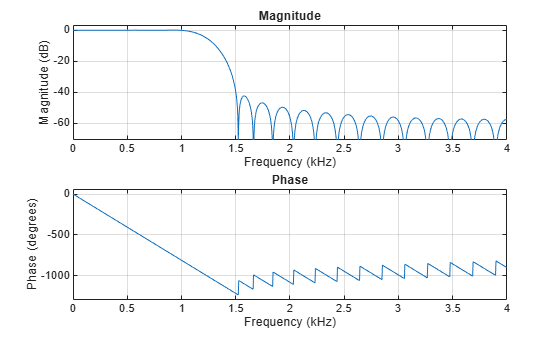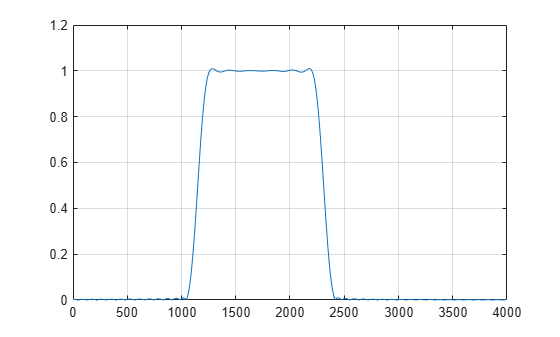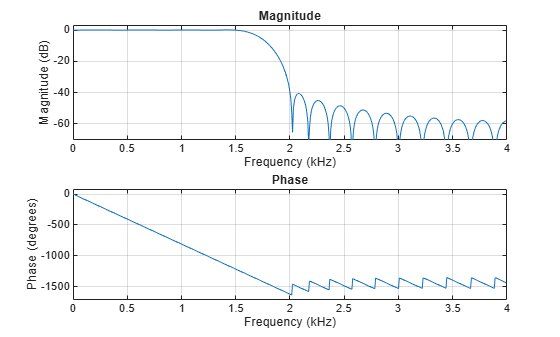# kaiserord

Kaiser window FIR filter design estimation parameters

## Syntax

``[n,Wn,beta,ftype] = kaiserord(f,a,dev)``
``[n,Wn,beta,ftype] = kaiserord(f,a,dev,fs)``
``c = kaiserord(f,a,dev,fs,'cell')``

## Description

````[n,Wn,beta,ftype] = kaiserord(f,a,dev)` returns a filter order `n`, normalized frequency band edges `Wn`, and a shape factor `beta` that specify a Kaiser window for use with the `fir1` function. To design an FIR filter `b` that approximately meets the specifications given by `f`, `a`, and `dev`, use ```b = fir1(n,Wn,kaiser(n+1,beta),ftype,'noscale')```.```

example

````[n,Wn,beta,ftype] = kaiserord(f,a,dev,fs)` uses a sample rate `fs` in Hz.```

example

````c = kaiserord(f,a,dev,fs,'cell')` returns a cell array whose elements are the parameters to `fir1`.```

## Examples

collapse all

Design a lowpass filter with passband defined from 0 to 1 kHz and stopband defined from 1500 Hz to 4 kHz. Specify a passband ripple of 5% and a stopband attenuation of 40 dB.

```fsamp = 8000; fcuts = [1000 1500]; mags = [1 0]; devs = [0.05 0.01]; [n,Wn,beta,ftype] = kaiserord(fcuts,mags,devs,fsamp); hh = fir1(n,Wn,ftype,kaiser(n+1,beta),'noscale'); freqz(hh,1,1024,fsamp)```Design an odd-length bandpass filter. Note that odd length means even order, so the input to `fir1` must be an even integer.

```fsamp = 8000; fcuts = [1000 1300 2210 2410]; mags = [0 1 0]; devs = [0.01 0.05 0.01]; [n,Wn,beta,ftype] = kaiserord(fcuts,mags,devs,fsamp); n = n + rem(n,2); hh = fir1(n,Wn,ftype,kaiser(n+1,beta),'noscale'); [H,f] = freqz(hh,1,1024,fsamp); plot(f,abs(H)) grid```Design a lowpass filter with a passband cutoff of 1500 Hz, a stopband cutoff of 2000 Hz, a passband ripple of 0.01, a stopband ripple of 0.1, and a sample rate of 8000 Hz. Design an equivalent filter using the `'cell'` option.

```fs = 8000; [n,Wn,beta,ftype] = kaiserord([1500 2000],[1 0],... [0.01 0.1],fs); b = fir1(n,Wn,ftype,kaiser(n+1,beta),'noscale'); c = kaiserord([1500 2000],[1 0],[0.01 0.1],fs,'cell'); bcell = fir1(c{:}); hfvt = fvtool(b,1,bcell,1,'Fs',fs); legend(hfvt,'b','bcell')```## Input Arguments

collapse all

Band edges, specified as a vector. The length of `f` is `2*length(a)-2`.

Band amplitude, specified as a vector. The amplitude is specified on the bands defined by `f`. Together, `f` and `a` define a piecewise-constant response function.

Maximum allowable deviation, specified as a vector. `dev` is a vector the same size as `a` that specifies the maximum allowable deviation between the frequency response of the output filter and its band amplitude, for each band. The entries in `dev` specify the passband ripple and the stopband attenuation. Specify each entry in `dev` as a positive number, representing absolute filter gain (unit-less).

Sample rate, specified as a positive scalar measured in Hz. If you do not specify the argument `fs`, or if you specify it as the empty vector `[]`, the sample rate defaults to 2 Hz, and the Nyquist frequency is 1 Hz. Use this syntax to specify band edges scaled to a particular application's sample rate. The frequency band edges in `f` must be from 0 to `fs`/2.

## Output Arguments

collapse all

Filter order, returned as a positive integer.

Normalized frequency band edges, returned as a real vector.

Shape factor, returned as a positive real scalar. The parameter `beta` affects the sidelobe attenuation of the Fourier transform of the window.

Filter type, intended for use with `fir1`, returned as:

• `'low'` — lowpass filter with cutoff frequency `Wn`.

• `'high'` — highpass filter with cutoff frequency `Wn`.

• `'bandpass'` — bandpass filter if `Wn` is a two-element vector.

• `'stop'` — bandstop filter if `Wn` is a two-element vector.

• `'DC-0'` — the first band of a multiband filter is a stopband.

• `'DC-1'` — the first band of a multiband filter is a passband.

FIR parameters, returned as a cell array.

## Tips

• Be careful to distinguish between the meanings of filter length and filter order. The filter length is the number of impulse response samples in the FIR filter. Generally, the impulse response is indexed from n = 0 to n = L – 1, where L is the filter length. The filter order is the highest power in a Z-transform representation of the filter. For an FIR transfer function, this representation is a polynomial in z, where the highest power is zL–1 and the lowest power is z0. The filter order is one less than the length (L – 1) and is also equal to the number of zeros of the z polynomial.

• If, in the vector `dev`, you specify unequal deviations across bands, the minimum specified deviation is used, since the Kaiser window method is constrained to produce filters with minimum deviation in all of the bands.

• In some cases, `kaiserord` underestimates or overestimates the order `n`. If the filter does not meet the specifications, try a higher order such as `n+1`, `n+2`, and so on, or a try lower order.

• Results are inaccurate if the cutoff frequencies are near 0 or the Nyquist frequency, or if `dev` is large (greater than 10%).

## Algorithms

Given a set of specifications in the frequency domain, `kaiserord` estimates the minimum FIR filter order that will approximately meet the specifications. `kaiserord` converts the given filter specifications into passband and stopband ripples and converts cutoff frequencies into the form needed for windowed FIR filter design.

`kaiserord` uses empirically derived formulas for estimating the orders of lowpass filters, as well as differentiators and Hilbert transformers. Estimates for multiband filters (such as bandpass filters) are derived from the lowpass design formulas.

The design formulas that underlie the Kaiser window and its application to FIR filter design are

`$\beta =\left\{\begin{array}{ll}0.1102\left(\alpha -8.7\right),\hfill & \alpha >50\hfill \\ 0.5842{\left(\alpha -21\right)}^{0.4}+0.07886\left(\alpha -21\right),\hfill & 21\le \alpha \le 50\hfill \\ 0,\hfill & \alpha <21\hfill \end{array}$`

where α = –20log10δ is the stopband attenuation expressed in decibels, and

`$n=\frac{\alpha -7.95}{2.285\left(\Delta \omega \right)}$`

where n is the filter order and Δω is the width of the smallest transition region.

 Digital Signal Processing Committee of the IEEE Acoustics, Speech, and Signal Processing Society, eds. Selected Papers in Digital Signal Processing. Vol. II. New York: IEEE Press, 1976.

 Kaiser, James F. “Nonrecursive Digital Filter Design Using the I0-Sinh Window Function.” Proceedings of the 1974 IEEE International Symposium on Circuits and Systems. 1974, pp. 20–23.

 Oppenheim, Alan V., Ronald W. Schafer, and John R. Buck. Discrete-Time Signal Processing. Upper Saddle River, NJ: Prentice Hall, 1999.